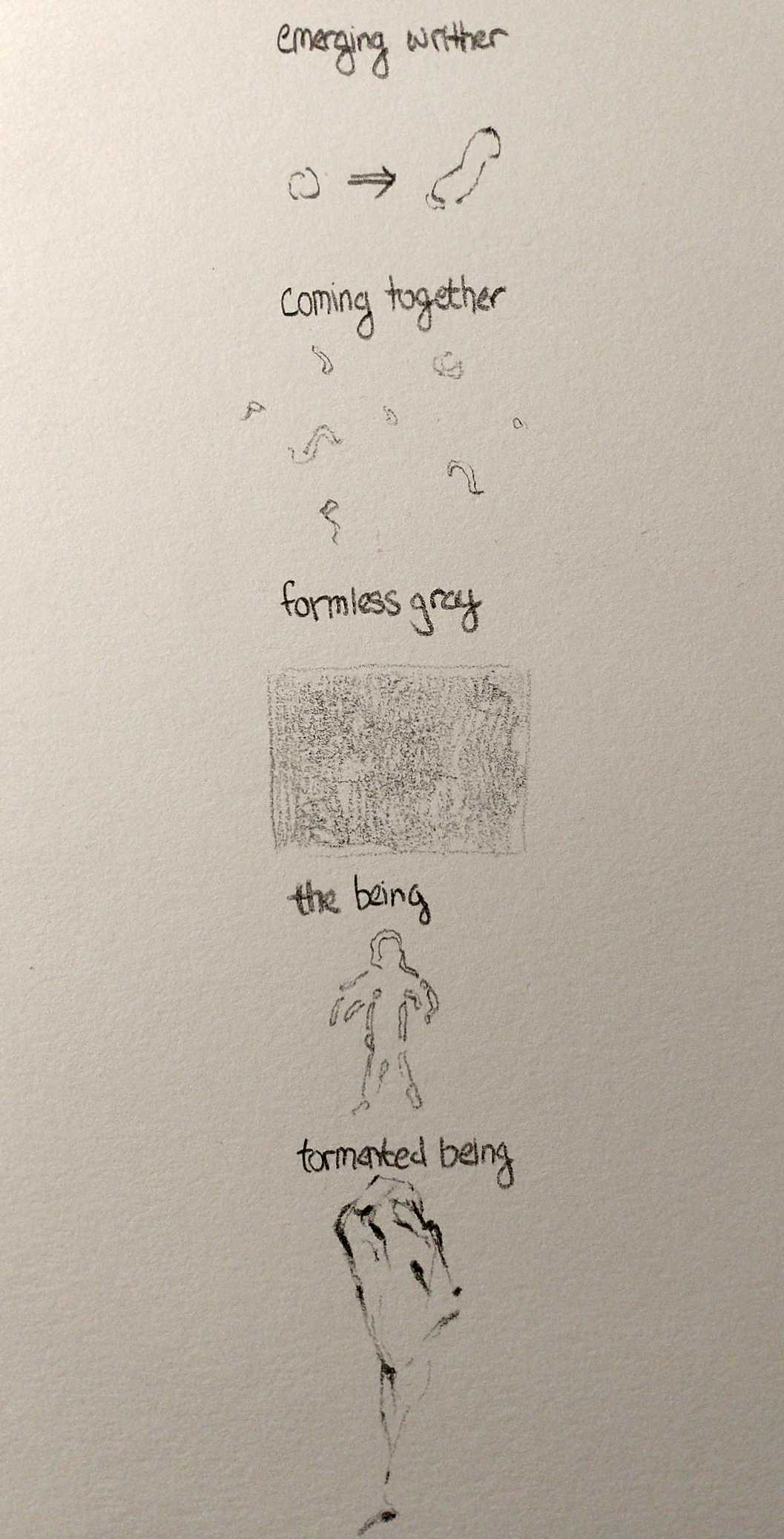# creatyde-AnimatedLoop```// Using the template provided by Dr. Golan Levin // Thanks to OpenCV made by Greg Borenstein import gab.opencv.*;   //===================================================   // Global variables. int nFramesInLoop = 100; int nElapsedFrames; boolean bRecording;   PImage img; // reference image for contours PImage backs[]; // background image array int numBack = 5; // number of background images int backChange = 3; // duration of each background image OpenCV opencv; ArrayList contours; ArrayList pos; // variable to determine direction of general contour movement boolean alt;   //===================================================   void setup() {   size (600, 600); bRecording = false; nElapsedFrames = 0; stroke(255); fill(255); strokeWeight(1.3);   // creating background images // https://forum.processing.org/two/discussion/13712/ // how-to-add-noise-to-the-image-being-drawn backs = new PImage[numBack]; for (int k = 0; k &lt; numBack; k++) { background(200); loadPixels(); for (int i = 0; i &lt; width; i+=2) { for (int j = 0; j &lt; height; j+=2) { float gray = 2*random(-1, 1); color c = 10*color(2*random(-1,1)+gray, 2*random(-1,1)+gray, 2*random(-1,1)+gray); pixels[i+j*width] += c; pixels[i+(j+1)*width] += c; pixels[(i+1)+j*width] += c; pixels[(i+1)+(j+1)*width] += c; } } updatePixels(); String name = "background" + k + ".jpg"; save(name); backs[k] = loadImage(name); }   // setup image img = loadImage("image.png"); img.resize(250, 250); opencv = new OpenCV(this, img); contours = opencv.findContours();   // setup contours pos = new ArrayList(); int i = 0; for (Contour c : contours) { ArrayList arr = c.getPoints(); pos.add(arr.get(0).copy()); for (int k = 0; k &lt; c.numPoints(); k++) { arr.get(k).x = arr.get(k).x - pos.get(i).x; arr.get(k).y = arr.get(k).y - pos.get(i).y; } i++; } // initialize direction of contours alt = false; }   //===================================================   // Thanks to Dr. Golan Levin for Pattern-master function float function_HannWindow (float x) { // http://en.wikipedia.org/wiki/Window_function float y = 0.5 * (1.0 - cos(TWO_PI*x)); return y; }   void draw() {   // Compute a percentage (0...1) representing // where we are in the loop. float percentCompleteFraction = 0; if (bRecording) { percentCompleteFraction = (float) nElapsedFrames / (float)nFramesInLoop; } else { percentCompleteFraction = (float) (frameCount % nFramesInLoop) / (float)nFramesInLoop; }   // Render the design, based on that percentage. renderMyDesign (percentCompleteFraction);   // If we're recording the output, save the frame to a file. if (bRecording) { saveFrame("output/creatyde-loop-" + nf(nElapsedFrames, 4)   + ".png"); nElapsedFrames++; if (nElapsedFrames &gt;= nFramesInLoop) { bRecording = false; } } }   //===================================================   void renderMyDesign (float percent) { // pre-saved noise image image(backs[(frameCount/backChange)%numBack], 0, 0); if (frameCount % nFramesInLoop/2 == 0) { alt = !alt; } // draw contours of the face pushMatrix(); translate(width/3.4, height/3.4); // true position int i = 0; float f = function_HannWindow(percent); float co = 1-sqrt(f); float t = 300*co; if (alt) t *= -1; float s = 1-abs(co); for (Contour c : contours) { pushMatrix(); rotate(radians(360/contours.size()*i)); translate(t, 0); rotate(-radians(360/contours.size()*i)); translate(pos.get(i).x+random(-5,5), pos.get(i).y+random(-5,5)); rotate(co); scale(s+random(-0.1,0.1)); c.draw(); popMatrix(); i++; } popMatrix(); }   //===================================================   void keyPressed() { bRecording = true; nElapsedFrames = 0; }```